# The solution of cvx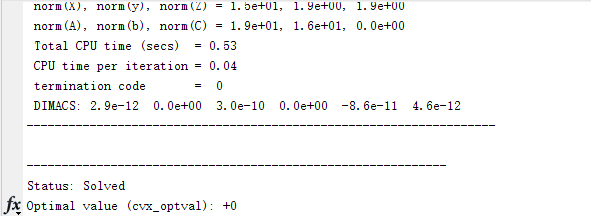The solution of cvx is always 0, and each run is exactly the same, and the generated power P does not meet the constraints.

Show your complete output, not just part of it. and maybe post your code out in markdown format, so someone can reproduce your problem.

function [Q_,P_] = CVX_theta_Optimal(M,P_max,K,Z,v,q,p)

cvx_begin
cvx_quiet false;
variable Q(M+1,M+1) hermitian semidefinite

``````expression F(K)
expression G(K)
expression P(K)

for i=1:K
if i == 1
s_inter = 0;
G_tmp = 0;
else
for j=1:i-1
s_inter = p(j).*(trace(Z(:,:,j)*q)+ square(abs(v(:,j)))) + s_inter;
G_tmp = G_tmp + Z(:,:,j)';
end
end
F(i) = -log(real(trace(Z(:,:,i)*Q)+abs(v(:,i)).^2))/log(2);
end
minimize sum(P)
subject to
diag(Q)  == 1 ;
for i = 1:K
0<=P(i)<=P_max;
P(i) = real(G(i))+F(i) ;
end
``````

cvx_end
Q_ = Q;
P_ = P ;

end
This is my code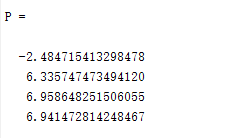This is the output of power；It is always one and does not satisfy the constraints.

Thank you so much for helping me take a look.

P(i) = real(G(i))+F(i) should be P(i) == real(G(i))+F(i). pls read the,cvx users’ guide.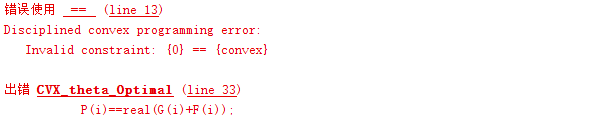This error is like this, P is an expression about Q, it is not a number.

function [Q_,P_] = CVX_theta_Optimal(M,P_max,K,Z,v,q,p,BER)

cvx_begin
cvx_quiet false;
variable Q(M+1,M+1) hermitian semidefinite
variable P(K)

``````expression F(K)
expression G(K)
expression A(K)

for i=1:K
if i == 1
s_inter = 0;
G_tmp = 0;
else
for j=1:i-1
s_inter = p(j).*(trace(Z(:,:,j)*q)+ norm((v(:,j)))) + s_inter;
G_tmp = G_tmp + Z(:,:,j)';
end
end
F(i) = -log(real(trace(Z(:,:,i)*Q)+norm(v(:,i))))/log(2);
end
``````

% expression N
%
%
minimize sum§
subject to
for i=1:K
0<P(i)<=P_max;
end
diag(Q) == 1 ;
for i = 1:K
A(i) = 2.^((real(real(G(i))+F(i))+log(power((5.5+sqrt(1/200)*qfuncinv(BER)/log(2)),2)-1)/log(2)));
A(i)<=P(i);
end
% N = 0;

cvx_end
I change my code to this ,but this solution is very small,it not satisfy this constraint.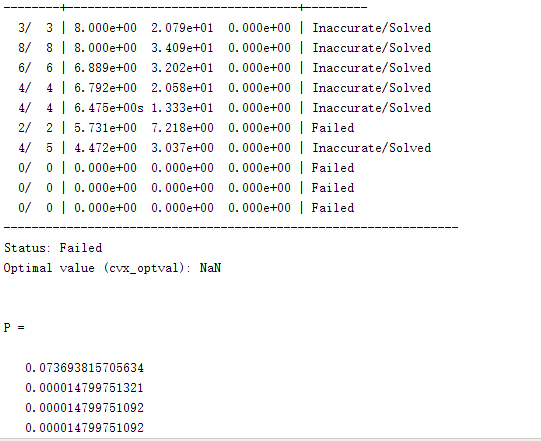function [Q_,P_] = CVX_theta_Optimal(M,P_max,K,Z,v,q,p,BER)

cvx_begin
cvx_quiet false;
variable Q(M+1,M+1) hermitian semidefinite
variable P(K)
expression F(K)
expression G(K)
for i=1:K
if i == 1
s_inter = 0;
G_tmp = 0;
else
for j=1:i-1
s_inter = p(j).(trace(Z(:,:,j)q)+ norm((v(:,j)))) + s_inter;
G_tmp = G_tmp + Z(:,:,j)’;
end
end
(s_inter+1);
G(i) = -rel_entr(1,real(s_inter+1))+2
F(i) = -(-rel_entr(1,(real(trace(Z(:,:,i)Q)+norm(v(:,i))))))/log(2);
end
% expression N
% N = norm_nuc(Q)-norm(q)+2
minimize P(1)+P(2)+P(3)+P(4)
subject to
for i=1:K
0<=P(i)<=P_max;
end
diag(Q) == 1 ;
for i = 1:K
P(i)-exp(log(2)
(log(2.^(2+(sqrt(1/200)*qfuncinv(BER)/log(2)))-1)/log(2)+real(real(G(i))+F(i))))>=0;
end
% N = 0;

cvx_end
%Q
P
Q_ = Q;
P_ = P;

end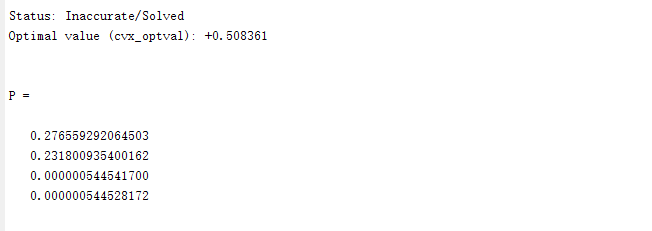I change my code to this.Although the optimal value can be generated, it is still not satisfied with the constraint, and the gap is very large. Sometimes NAN also occur.Can you help me see what is the problem?

Thank you !Hope you can help me take a look at this problem.

You haven’t shown us the output. We don’t even know what solution method was used. Note that constrains are not satisfied exactly, but only to within a tolerance. And CVX reported that your problem was solved inaccurately. It is possible that your input data is not well-scaled.

Sorry，This is my code and output.
function [Q_,P_] = CVX_theta_Optimal(M,P_max,K,Z,v,q,p,BER)

cvx_solver mosek
cvx_begin
cvx_quiet false;
variable Q(M+1,M+1) hermitian semidefinite

``````expression F(K)
expression G(K)
expression P(K)

for i=1:K
if i == 1
s_inter = 0;
G_tmp = 0;
else
s_inter = 0;
G_tmp = 0;
for j=1:i-1
s_inter = p(j).*(trace(Z(:,:,j)*q)+ sum(abs(v(:,i)).^2)) + s_inter;
G_tmp = G_tmp + Z(:,:,j).';
end
end
F(i) = -(-rel_entr(1,(real(trace(Z(:,:,i)*Q)+sum(abs(v(:,i)).^2)))))/log(2);
end
%expression N
minimize sum(P)
subject to
diag(Q) == 1 ;

for i=1:K
0<=P(i)<=P_max;
end

for i=1:K
P(i)-exp(log(2)*(log(2.^(1+(sqrt(1/200)*qfuncinv(BER)/log(2)))-1)/log(2)+real(G(i)+F(i))))>=0;
end

%N = 0;
``````

cvx_end
%Q
P
Q_ = Q;
P_ = P;

## Cones | Errors | Mov/Act | Centering Exp cone Poly cone | Status --------±--------------------------------±-------- 0/ 2 | 8.000e+00 6.868e+02 3.299e+43 | Infeasible

Status: Infeasible
Optimal value (cvx_optval): +Inf

P =

NaN
NaN
NaN
NaN

I don’t know why this problem occurs. I don’t know how to modify my code to make it run normally. Please help me, thank you!!!

Sorry，This is my code and output.